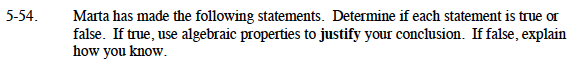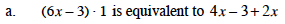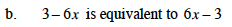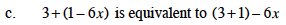Home > CAAC > Chapter 5 > Lesson 5.1.5 > Problem5-54

5-54.
1. Marta has made the following statements. Determine if each statement is true or false. If true, use algebraic properties to justify your conclusion. If false, explain how you know. Homework Help ✎

1. (6x − 3) · 1 is equivalent to 4x − 3 + 2x

2. 3 − 6x is equivalent to 6x − 3

3. 3 + (1 − 6x) is equivalent to (3 + 1) − 6xTrue. Multiplicative Identity.False. Subtraction is not commutative.True. Associative Property of Addition.